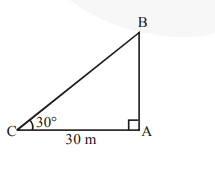# The angle of elevation of the top of a tower from a point on the ground,Question:

The angle of elevation of the top of a tower from a point on the ground, which is 30 m away from the foot of the tower, is 30°. Find the height of the tower.

Solution:

In right ABC, AB = height of the tower and point C is 30m away from the foot of the tower,

$\therefore \quad \mathrm{AC}=30 \mathrm{~m}$$\operatorname{Now} \frac{\mathbf{A B}}{\mathbf{A C}}=\tan 30^{\circ}$

$\Rightarrow \frac{\mathbf{h}}{\mathbf{3 0}}=\frac{1}{\sqrt{\mathbf{3}}}$

$\left[\because \tan 30^{\circ}=\frac{\mathbf{1}}{\sqrt{\mathbf{3}}}\right]$

$\Rightarrow \mathrm{h}=\frac{\mathbf{3 0}}{\sqrt{3}}=\frac{\mathbf{3 0}}{\sqrt{3}} \times \frac{\sqrt{3}}{\sqrt{3}}=10 \sqrt{3}$

Thus, the required height of the tower is $10 \sqrt{3} \mathrm{~m}$